# Lesson 3 6 Implicit Differentiation Objectives Use implicit

• Slides: 8Lesson 3 -6 Implicit DifferentiationObjectives • Use implicit differentiation to solve for dy/dx in given equations • Use inverse trig rules to find the derivatives of inverse trig functions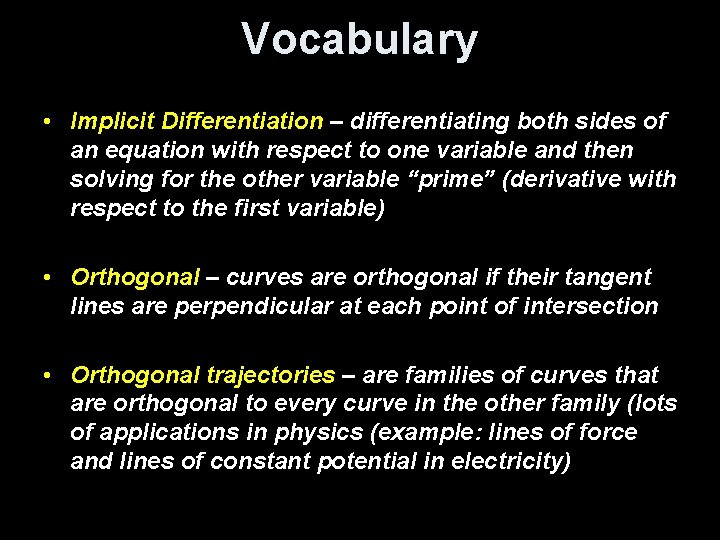Vocabulary • Implicit Differentiation – differentiating both sides of an equation with respect to one variable and then solving for the other variable “prime” (derivative with respect to the first variable) • Orthogonal – curves are orthogonal if their tangent lines are perpendicular at each point of intersection • Orthogonal trajectories – are families of curves that are orthogonal to every curve in the other family (lots of applications in physics (example: lines of force and lines of constant potential in electricity)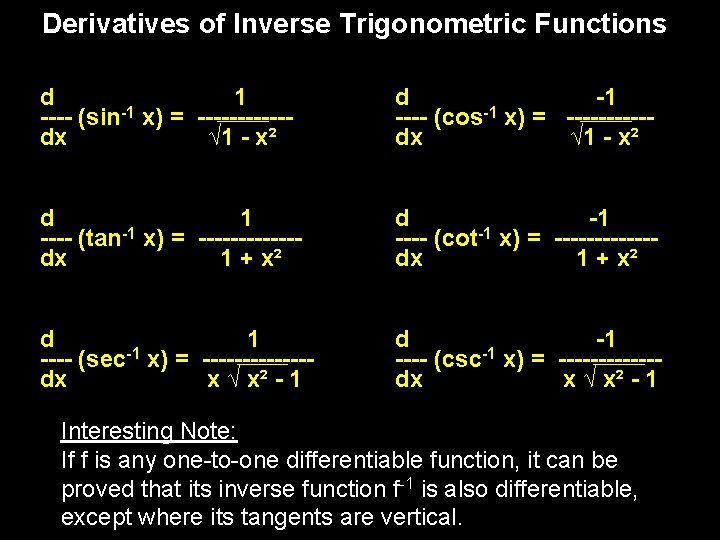Derivatives of Inverse Trigonometric Functions d 1 -1 ---- (sin x) = ------dx √ 1 - x² d -1 -1 ---- (cos x) = -----dx √ 1 - x² d 1 -1 ---- (tan x) = ------dx 1 + x² d -1 -1 ---- (cot x) = ------dx 1 + x² d 1 -1 ---- (sec x) = -------dx x √ x² - 1 d -1 -1 ---- (csc x) = ------dx x √ x² - 1 Interesting Note: If f is any one-to-one differentiable function, it can be proved that its inverse function f-1 is also differentiable, except where its tangents are vertical.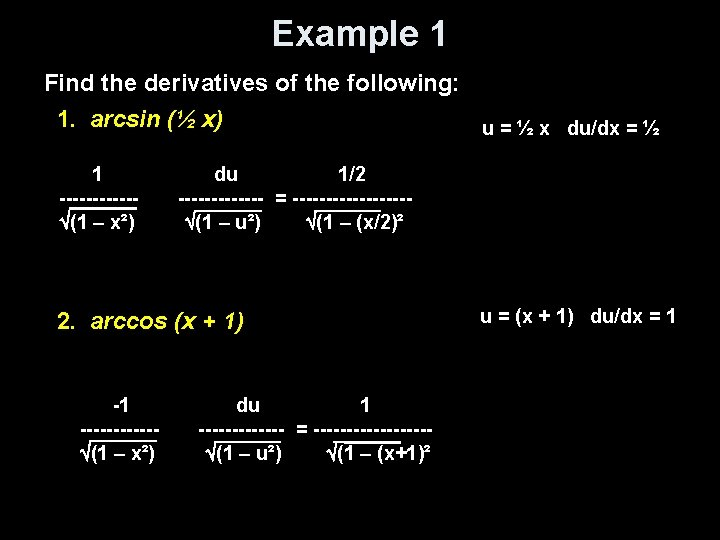Example 1 Find the derivatives of the following: 1. arcsin (½ x) u = ½ x du/dx = ½ 1 ------ (1 – x²) du 1/2 ------- = --------- (1 – u²) (1 – (x/2)² 2. arccos (x + 1) -1 ------ (1 – x²) du 1 ------- = --------- (1 – u²) (1 – (x+1)² u = (x + 1) du/dx = 1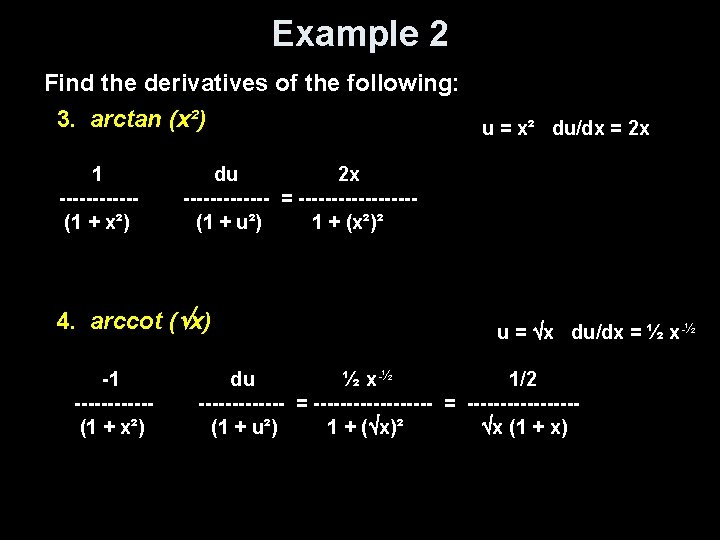Example 2 Find the derivatives of the following: 3. arctan (x²) u = x² du/dx = 2 x 1 ------(1 + x²) du 2 x ------- = ---------(1 + u²) 1 + (x²)² 4. arccot ( x) -1 ------(1 + x²) u = x du/dx = ½ x-½ du ½ x -½ 1/2 ------------- = -----------(1 + u²) 1 + ( x)² x (1 + x)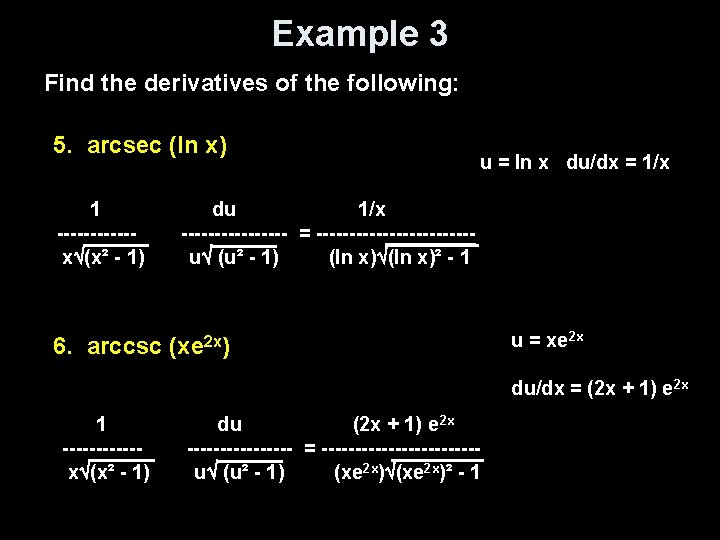Example 3 Find the derivatives of the following: 5. arcsec (ln x) 1 ------x (x² - 1) u = ln x du/dx = 1/x du 1/x -------- = ------------u (u² - 1) (ln x)² - 1 6. arccsc (xe 2 x) u = xe 2 x du/dx = (2 x + 1) e 2 x 1 ------x (x² - 1) du (2 x + 1) e 2 x -------- = ------------u (u² - 1) (xe 2 x)² - 1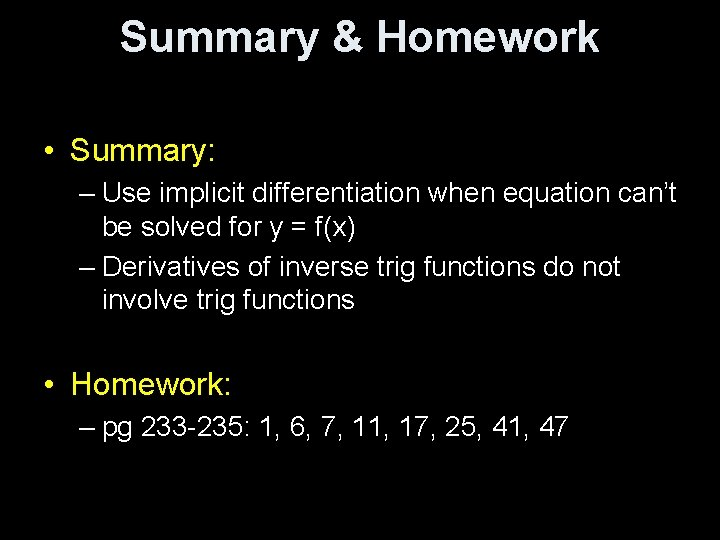Summary & Homework • Summary: – Use implicit differentiation when equation can’t be solved for y = f(x) – Derivatives of inverse trig functions do not involve trig functions • Homework: – pg 233 -235: 1, 6, 7, 11, 17, 25, 41, 47